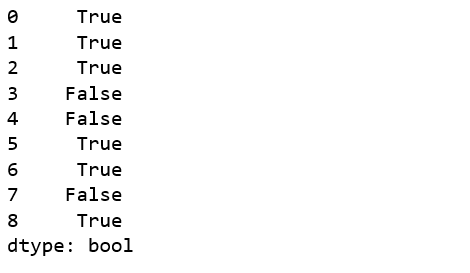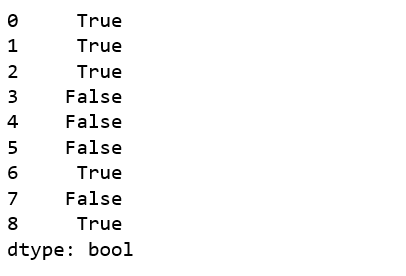# Python | Pandas Series.le()

Python is a great language for doing data analysis, primarily because of the fantastic ecosystem of data-centric Python packages. Pandas is one of those packages and makes importing and analyzing data much easier.

Pandas `series.le()` is used to compare every element of Caller series with passed series. It returns True for every element which is Less than or Equal to the element in passed series.

Note: The results are returned on the basis of comparison caller series <= other series.

Syntax: Series.le(other, level=None, fill_value=None, axis=0)

Parameters:
other: other series to be compared with
level: int or name of level in case of multi level
fill_value: Value to be replaced instead of NaN
axis: 0 or ‘index’ to apply method by rows and 1 or ‘columns’ to apply by columns.

Return type: Boolean series

Example #1: NaN handling

In this example, two series are created using `pd.Series()`. The series contains some Null values and some equal values at same indices too. The series are compared using `le()` method and 10 is passed to fill_value parameter to replace NaN values by 10.

 `# importing pandas module   ` `import` `pandas as pd   ` `   `  `# importing numpy module  ` `import` `numpy as np  ` `   `  `# creating series 1  ` `series1 ``=` `pd.Series([``11``, ``0``, ``2``, ``43``, ``9``, ``27``, np.nan, ``10``, np.nan])  ` `   `  `# creating series 2  ` `series2 ``=` `pd.Series([``16``, np.nan, ``2``, ``23``, ``5``, ``40``, ``54``, ``3``, ``19``])  ` ` `  `# NaN replacement ` `replace_nan ``=` `10` ` `  `# calling and returning to result variable ` `result ``=` `series1.le(series2, fill_value ``=` `replace_nan) ` ` `  `# display  ` `result  `

Output:
As shown in output, True was returned wherever value in caller series was less than or Equal value in passed series. Also it can be seen Null values were replaced by 10 and the comparison was made using that value.Example #2: Calling on Series with str objects

In this example, two series are created using` pd.Series()`. The series contains some string values too. In case of strings, the comparison is made with their ASCII values.

 `# importing pandas module   ` `import` `pandas as pd   ` `   `  `# importing numpy module  ` `import` `numpy as np  ` `   `  `# creating series 1  ` `series1 ``=` `pd.Series([``'A'``, ``0``, ``'c'``, ``43``, ``9``, ``'e'``, np.nan, ``'x'``, np.nan])  ` `   `  `# creating series 2  ` `series2 ``=` `pd.Series([``'v'``, np.nan, ``'c'``, ``23``, ``5``, ``'D'``, ``54``, ``'p'``, ``19``])  ` ` `  `# NaN replacement ` `replace_nan ``=` `10` ` `  `# calling and returning to result variable ` `result ``=` `series1.le(series2, fill_value ``=` `replace_nan) ` ` `  `# display  ` `result  `

Output:
As it can be seen in output, in case of strings, the comparison was made using their ASCII values.My Personal Notes arrow_drop_upCheck out this Author's contributed articles.

If you like GeeksforGeeks and would like to contribute, you can also write an article using contribute.geeksforgeeks.org or mail your article to contribute@geeksforgeeks.org. See your article appearing on the GeeksforGeeks main page and help other Geeks.

Please Improve this article if you find anything incorrect by clicking on the "Improve Article" button below.

Improved By : Akanksha_Rai

Article Tags :

Be the First to upvote.

Please write to us at contribute@geeksforgeeks.org to report any issue with the above content.